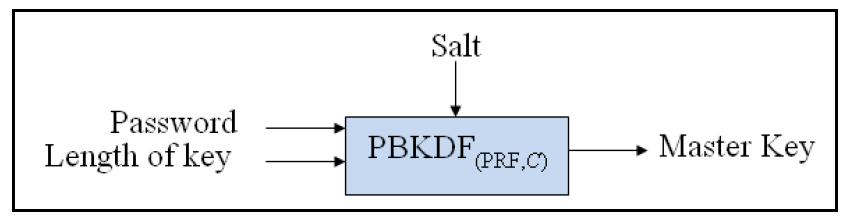# CISSP PRACTICE QUESTIONS – 20191209Alice and Bob are students with a major in Computer Science, taking the Cryptography course this semester. They turned in the homework of implementing a 128-bit cryptographic key generator graded in terms of entropy. Alice received an A, while Bob received a B. Why did the professor grade so?
A. Alice’s generates keys faster than Bob’s
B. The entropy values of Alice and Bob are 0.970950594 and 0.992774454 respectively
C.  Alice used mouse movements to generate randomness, while Bob used standard Operating System-level Application Programming Interface (API) functions
D. Alice’s key space is larger than Bob’s.

Kindly be reminded that the suggested answer is for your reference only. It doesn’t matter whether you have the right or wrong answer. What really matters is your reasoning process and justifications.

My suggested answer is D. Alice’s keyspace is larger than Bob’s.NIST SP 800-90B

# Entropy

Entropy is a measure of the disorder, randomness or variability in a closed system. An entropy value is between 0 and 1. The higher an entropy value is, the more unpredictable a key generator is.

# Random Bit Generator (RBG)

It’s common for a key generator to generate or derive a key based on a Random Bit Generator (RBG). In other words, the entropy of an RGB affects the keyspace of a key generator. “In cryptography, an algorithm’s key space refers to the set of all possible permutations of a key.” (Wikipedia)

A noise source is the root of security for the entropy source and for the RBG as a whole. E.g. mouse movements or API functions.

# PBKDF (Password-Based Key Derivation Functions)

The salt in the PBKDF shall be generated using an approved Random Bit Generator according to NIST SP 800-132.NIST SP 800-132

# Summary

• The keyspace is affected by the key generation algorithm and the entropy of the RGB it depends on. A good key generator should have a larger keyspace than the other.
• The homework is graded in terms of entropy, so the speed of the key generation is not an option.
• Alice’s entropy value is 0.970950594 which is less than Bob’s 0.992774454. Bob’s key generator is more random or unpredictable than Alice.
• Both mouse movements and API functions are noise sources. Both of them affect the entropy of the RGB, but the entropy is unknown. Moreover, the keyspace may be affected by the implementation of algorism.